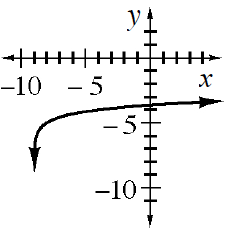### Home > INT3 > Chapter 12 > Lesson 12.2.1 > Problem12-89

12-89.

Graph $y=\log_5(x+9)-5$. Homework Help ✎1. What are the domain and range of the graph?

D: $x<−9$ R: all real numbers

2. Where are the intercepts and the locator point?

let $x = 0$ to find the $y$-intercept and
$y = 0$ to find the $x$-intercept.

3. Write the equation of the inverse.

$y=5^{(x+5)}−9$

4. What are the domain and range of the inverse?

If you know the domain and range of the function you also know it for the inverse.